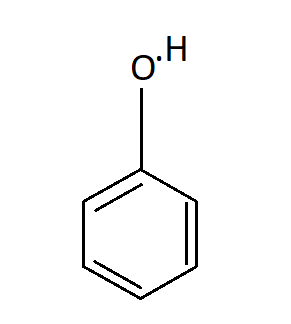Melting point of phenol is:$A.\;{95^ \circ }C$$B.\;{43^ \circ }C$$C.\;{20^ \circ }C$$D.\;{10^ \circ }C$Verified
148.5k+ views
Hint: An organic compound-Phenol is appreciably soluble in water and because of its commercial importance many methods have been developed for its production. They are generally colourless solids or liquids and have relatively low melting point.

Phenol is also known as carbolic acid or Hydroxybenzene or Phenic acid. And its molecular formula is ${C_6}{H_5}OH$. It is an aromatic organic compound. It is a white crystalline solid that is volatile. The molecule of Phenol consists of a phenyl group $( - {C_6}{H_5})$which is bonded to a hydroxyl group $( - OH)$. It requires careful handling because it is mildly acidic and can cause chemical burns. In aqueous solution the pH range is 8 – 12 pH. And its melting point is ${43^ \circ }C$Hence our answer is B. ${43^ \circ }C$